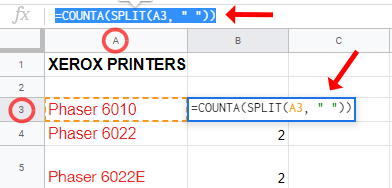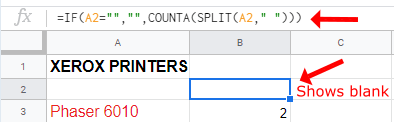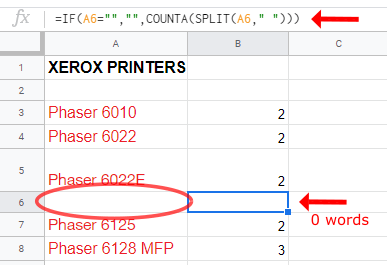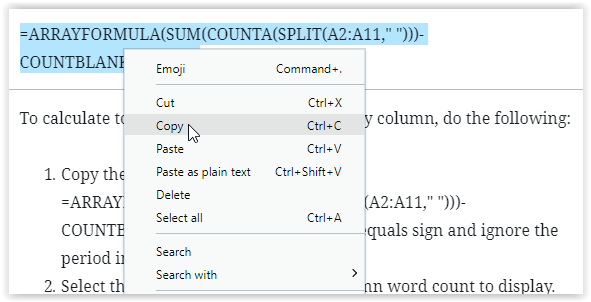# How To Get Word Count in Google Sheets

While Google Sheets is primarily made to be used with numbers, words are an important part of any spreadsheet. You need words to calculate, verify, and keep track of what each data point represents. Sometimes, you have word count limits per cell. Labels, months, days, products—they all need specific words for the list too. Formulas play a big role in spreadsheet functionality, and word counts in Google Sheets require it. Just like using IF/THEN statements for datasets in Google Sheets, word counts use COUNTA, SPLIT, SUM, ARRAYFORMULA, and more. So, what do the statements provide for Google sheets? Here’s more information.

Google Docs allows you to easily check the list of words in any given document, giving you a count for both the entire document and the content you have highlighted using your mouse. It is really easy to figure out how long a Google Doc document is in terms of word length, but unfortunately (at first glance), that traditional word count option is not present in Google Sheets. If you are looking for a way to figure out how many words are in your Google Sheets spreadsheet versus Google Docs, there is an easy workaround that anyone can use. Let us take a look.

## Word Count Options for Google Sheets

Google Sheets spreadsheets have the ability to count words within the document, but it is not a clickable action as it is in Docs. While there is no official word count tool, Sheets does display a text count within specific columns, rows, and cells when using formulas.

### Counting Text per Cell in Google Sheets

Counting words per cell in Google Sheets has 2 different formulas based on specific needs. The first formula shown below calculates total word count per cell when there are no blank cells within the range specified, such as from A2 to A8. If you have any empty cells between your specified range, see the second formula below.

#### Option #1: Word Count per Cell with No Empty Cells in Specified Range

To preview word counts per cell when there are no empty cells in between, use the following instructions.

1. Highlight the blank cell where you want to display your results and paste the following formula: =COUNTA(SPLIT(A3, ” “)) where “A3” specifies the cell.2. Click in your display cell or hit Enter to apply the formula.As you can see above, cell A3 has two words. Here is the breakdown of the formula used, which was “=COUNTA(SPLIT(A3, ” “)).”

• COUNTA auto-counts the words in the cell.
• SPLIT counts everything separated by a space as an individual point of data (your content can be counted as a word, even if it’s just a number).
• A2 translates to Column, Row Number where “A” is the column and “2” is the row number, which totals the word count in the specified cell.

#### Option #2: Word Count per Cell with Empty Cells in Specified Range

To preview word counts per cell with some cells being empty within your specified range, use the following instructions.

1. Highlight the blank cell where you want to display your results and paste the following formula: =IF(A2=””,””,COUNTA(SPLIT(A2,” “))) where “A2” specifies the cell to be counted.2. Click in your display cell or hit Enter to apply the formula.In formula 2 above, using the “IF” command determines if there are empty cells, and if so, it does not count the cells as 1 word. Formula 1 above counts each empty cell as 1 word.### Counting Text per Column in Sheets

You could use the cell method to count each specific cell to receive a total word count, but as you can imagine for larger documents, this will take longer than you might want.

In order to properly provide the word count for your document using a much faster method, you can count your text in Sheet by using each column, instead of each cell.

The formula used for Google Sheets word count in columns also has two options, but the second one covers both calculations. Rather than waste time adding two different formulas (one for columns without blank cells and one for columns with them) you can use the following formula below.

=ARRAYFORMULA(SUM(COUNTA(SPLIT(A2:A11,” “)))-COUNTBLANK(A2:A11))

To calculate total Google Sheets word count by column, do the following:

1. Copy the formula shown above: =ARRAYFORMULA(SUM(COUNTA(SPLIT(A2:A11,” “)))-COUNTBLANK(A2:A11)). Start with the equals sign and ignore the period in the end when copying.2. Select the cell where you want the column word count to display.3. Right-click the text box at the top that shows the cell’s contents, and then choose “Paste as plain text.” This ensures that the correct font and characters get pasted.4. Edit the formula in the text box to reflect the correct cell range, then press enter to save it. Don’t click on another cell to save it or it may change your cell range.Yes, it is a more complex formula, but the method of using it is just as simple as you might expect from Google Sheets. The formula for column counts ignores blank cells (without counting them as 1) and counts the words in each column cell to deliver a total word count for the column range specified.

### ***

While it is unfortunate that your content can’t be automatically counted, as it can within Google Docs, it is not too hard to use the formula tool within Google Sheets to quickly and easily add content to your document and add up a certain word count. With just the quick application of a formula, you can access the data you want, whenever you want it.

Disclaimer: Some pages on this site may include an affiliate link. This does not effect our editorial in any way.

Todays Highlights